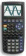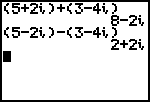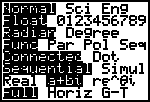Go to content
To divide two complex numbers, multiply by the complex conjugate of the denominator.

## Summary/BackgroundA note about graphic calculatorsA graphic calculator, for example the TI-83 or similar model, can work with complex numbers.First select MODE and move down to select a+bi:Then press 2nd QUIT and enter a complex number sum.You can get a better display of the maths by downloading special TeX fonts from jsMath. In the meantime, we will do the best we can with the fonts you have, but it may not be pretty and some equations may not be rendered correctly.

## Glossary

### complex number

A number of the form a+bi where i is the square root of -1, and a and b are real.

### conjugate

of a complex number a+bi is a-bi.

### denominator

the integer on the bottom of a fraction

### mode

the value in a set of discrete data that occurs most frequently

### union

The union of two sets A and B is the set containing all the elements of A and B.

### work

Equal to F x s, where F is the force in Newtons and s is the distance travelled and is measured in Joules.

Full Glossary List

## This question appears in the following syllabi:

SyllabusModuleSectionTopicExam Year
AQA A-Level (UK - Pre-2017)FP2Complex NumbersComplex algebra-
AQA AS Further Maths 2017Pure MathsComplex Number BasicsComplex Algebra-
AQA AS/A2 Further Maths 2017Pure MathsComplex Number BasicsComplex Algebra-
CBSE XI (India)AlgebraComplex Numbers and Quadratic EquationsImaginary unit-
CCEA A-Level (NI)FP1Complex NumbersComplex algebra-
CIE A-Level (UK)P3Complex NumbersComplex algebra-
Edexcel A-Level (UK - Pre-2017)FP1Complex NumbersComplex algebra-
Edexcel AS Further Maths 2017Core Pure MathsComplex NumbersComplex Algebra-
Edexcel AS/A2 Further Maths 2017Core Pure MathsComplex NumbersComplex Algebra-
I.B. Higher Level1Complex NumbersComplex algebra-
Methods (UK)M3Complex NumbersComplex algebra-
OCR A-Level (UK - Pre-2017)FP1Complex NumbersComplex algebra-
OCR AS Further Maths 2017Pure CoreComplex Number BasicsComplex Algebra-
OCR MEI AS Further Maths 2017Core Pure AComplex Number BasicsComplex Algebra-
OCR-MEI A-Level (UK - Pre-2017)FP1Complex NumbersComplex algebra-
Pre-U A-Level (UK)7Complex NumbersComplex algebra-